# Astronomy 12 - Spring 1999 (S.T. Myers)

## Solutions to Problem Set #8

Solutions:
1. The purpose of this problem is to illustrate the use of the Tully-Fisher relation for determining distances to galaxies, as well as computing mass-to-light ratios.

(a)
After plug-and-play, we find

MB = -21.83

(b)
The distance modulus is

B - MB = 34.05 = 5 log d - 5

which gives a distance of 64.57 Mpc to NGC 2639.

(c)
The relation gives

log R25 = 1.4357

or R25 = 27.27 kpc.

(d)
The sky brightness (for example in one square arcsecond) is larger by a factor of

Isky/I25 = 100.4(25-22) = 101.2 = 15.8

and thus you need to measure the galaxy light down to a level of 6% of the sky brightness.

(e)
Our mass is (remembering to convert km/s to m/s and kpc to m)

M = v2 R / G = 1.32 × 1042 kg = 6.62 × 1011 Msun

(f)
The B magnitude of the Sun is

MB,sun = MV,sun + ( B - V ) = 4.83 + 0.64 = 5.47

ie. the Sun is fainter in the blue band relative to an A0 star which sets the magnitude zero. Therefore

L / Lsun = 100.4(4.83 - MB) = 1010.66 = 4.61 × 1010

for NGC 2639 in B.

(g)
The mass-to-light ratio is

M / L = 6.62 × 1011 Msun / 4.61 × 1010 Lsun = 14.36 Msun / Lsun

This ratio is consistent what that for normal spiral galaxies of 2 - 20 (ZGS p.419).

2. We compute the sums (using a handy calculator)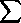(di /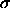i2) = 56.1082

andi-2 = 3.5485

giving

davg = 56.1082 / 3.5485 = 15.81 Mpc

for our best estimate for the Virgo distance.

The uncertainty in our estimate is therefored = 3.5485-1/2 = 0.53 Mpc

thus

dVirgo = 15.81 ± 0.53 Mpc

is our sample weighted average Virgo distance.

3. The Hubble constant is given by

H0 = v / d = 1136 km/s / 15.81 Mpc = 71.85 km/s/Mpc.

Note that the uncertainty in our derived H0 isH0 / H0 =d / d = 0.53 / 15.81 = 0.0335

(though this was not part of the question) and thus we have a 3.35% uncertainty or

H0 = 71.9 ± 2.4 km/s/Mpc

keeping a reasonable number of digits. Usually the systematic error in measurements will be greater than this (for example: all methods are based on Cepheid distances in our galaxy).

After correction for 168 km/s infall toward Virgo, the recession velocity is 1304 km/s giving

H0 = 82.5 ± 2.8 km/s/Mpc

which was a larger correction that the statistical uncertainty of our distance measurement! Note the uncertainty scales with the Hubble constant, since it is a ratio of the two numbers.

The Hubble time is then

1 / H0 = 1/82.5 s Mpc/km × 3.086 × 1019 km / Mpc = 3.74 × 1017 s = 11.85 Gyr

with again a 3.35% statistical uncertainty from the distance

tH = 11.85 ± 0.40 Gyr.

4. With a velocity dispersion of 666 km/s we get the virial mass of

Mvir = 1.54 × 1045 kg = 7.70 × 1014 Msun

rembering again to convert to MKS units.

To cross the diameter of the Virgo cluster

D = 3 Mpc × 3.086 × 1019 km/Mpc = 9.26 × 1019 km

at a velocity of 666 km/s would take

tcross = 1.39 × 1017 s = 4.4 Gyr

which is less than the Hubble time of 12 Gyr.

The Hubble velocity between opposite sides of the cluster is

vH = 3 Mpc × 82.5 km/s/Mpc = 248 km/s

which is 37% of the velocity dispersion of 666 km/s. Note that this is the same fraction as crossing time compared to the Hubble time, since this is the same calculation

vH /r = D H0 /r = (D/r) / (1/H0) = tcross / tH

as expected.

5. Using T = 7 × 107 K and R = 1.5 Mpc

(a)
The luminosity is given by

Lx = ( 4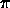R2 / 3 )ff = 1.5 × 1036 W

which givesff = 1.5 × 1036 W / 4.155 × 1068 m3 = 3.61 × 10-33 W/m3.

Substituting this in the formula for the free-free emissivity

ne = [ 3.61 × 10-33 / 1.42 × 10-40 ]1/2 [ 7 × 107 ]-1/4 = 55.12 m-3

with everything in the appropriate units.

(b)
If the IGM gas is hydrogen, and it is fully ionized (which it is) the hydrogen density will be equal to the electron density, so the mass density in the IGM will be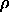= ne mH = 9.206 × 10-26 kg m-3

so the IGM mass is

Migm = 9.206 × 10-26 kg m-3 × 4.155 × 1068 m3 = 3.82 × 1043 kg = 1.91 × 1013 Msun.

(c)
Combining (b) and the results from problem 3, we get

Migm / Mvir = 1.91 × 1013 Msun/ 7.70 × 1014 Msun = 0.025

or 2.5% of the mass of the cluster is in the hot intergalactic medium.

(d)
The total mass-to-light ratio (using the virial mass) is

Mvir / L_V = 7.70 × 1014 Msun / 1.2 × 10^12 Lsun = 642

which is a very high value (and typical of clusters of galaxies). Using a spiral galaxy mass-to-light ratio (see Problem Set 7) of 10, we infer

Mgal ~ 10 L_V = 1.2 × 1013 Msun

which is 63% of the IGM gas mass. In more massive clusters than Virgo, like Coma, the galaxies contribute and even smaller fraction of the visible mass.

(e)
The hot electrons and protons form a gas, with an energy density of

uigm = 3 nigm k T / 2 = 3 ne k T

assuming the electrons and protons have the same energy. Then

uigm = 1.60 × 10-13 J m-3

which if it emits at the rate given in (a) will lose all its energy in a time

tcool = uigm /ff = 1.60 × 10-13 J m-3 / 3.61 × 10-33 W m-3 = 4.43 × 1019 s = 1.4 × 1012 yrs

which is much longer than the Hubble time.

6. We calculate the Eddington luminosity

LEd = 4G M c /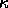= 1.26 × 1031 W · ( M / Msun )

using the electron scattering (Thomson) opacity. In terms of solar luminosities

LEd = 3.28 × 104 Lsun · ( M / Msun )

and thus 108 Msun gives 3.3 × 1012 Lsun ( 1.26 × 1039 W ), which is in the range of quasar luminosities.

Each kilogram of matter brought from "infinity" to a radius of q Schwarzschild radii will give up the change in gravitational energy (which is zero at R of infinity)

Ugr/m = G M / R = G M / ( q · 2GM/c2 ) = c2 / 2q

and thus delivers an appreciable fraction of its rest-mass energy mc2! For q=3, we get c2/6 per kg, or 1.5 × 1016 J/kg. Thus, to maintain the Eddington luminosity we calcluated above, we need to accrete mass at the rate

dm/dt = dM/dt = LEd / (Ugr/m) = ( 1.26 × 1039 J/s ) / ( 1.5 × 1016 J/kg ) = 8.4 × 1022 kg/s

or

dM/dt = ( 8.4 × 1022 kg/s) · ( 3.156 × 107 s/yr ) / ( 2 × 1030 kg/Msun ) = 1.33 Msun/yr

and thus our black hole just has to munch on a star every year or so (or one big star every few years)!

smyers@nrao.edu   Steven T. Myers